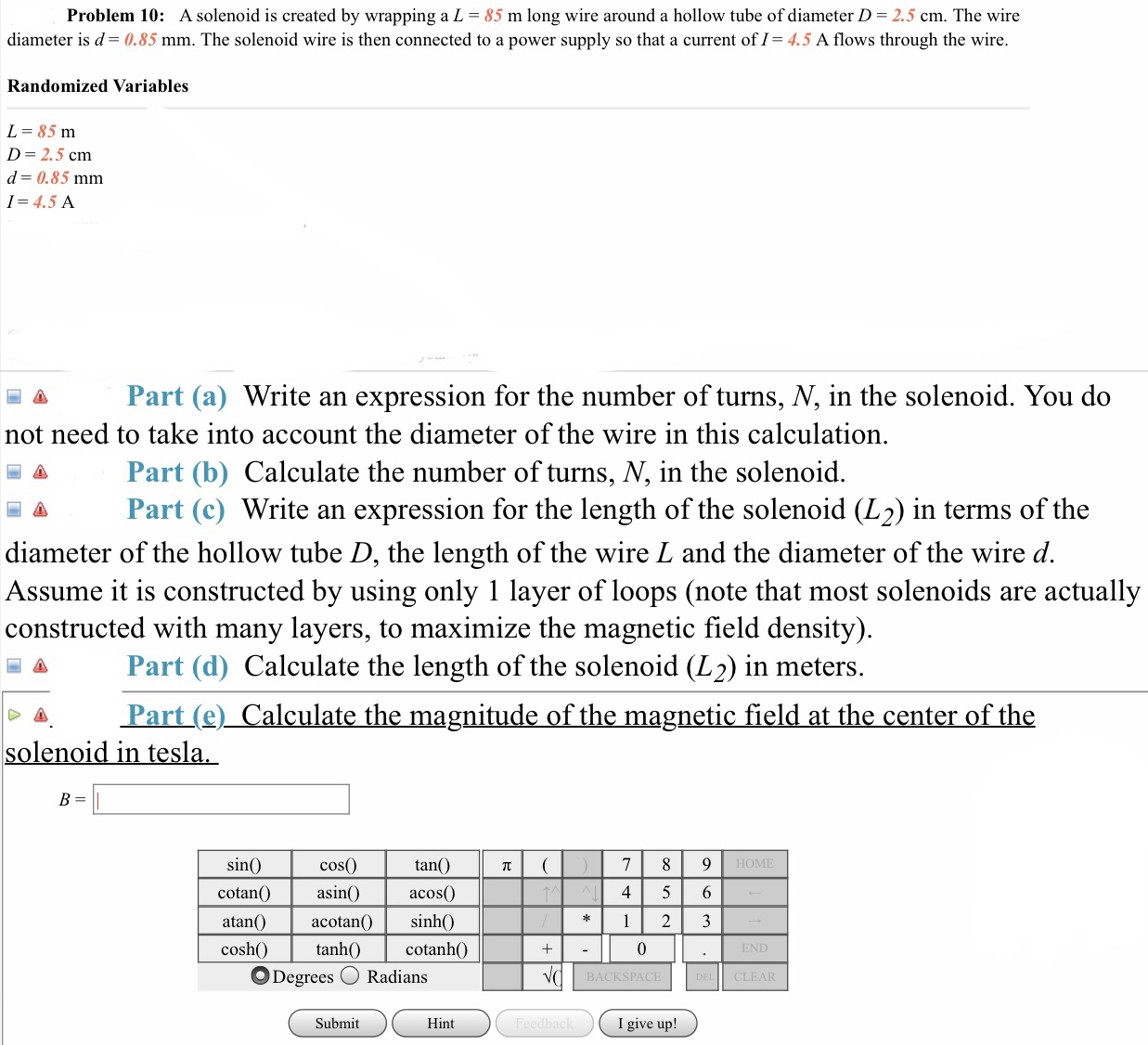# Problem 10:A solenoid is created by wrapping a L = 85 m long wire around a hollow tube of diameter D = 2.5 cm. The wirediameter is d= 0.85 mm. The solenoid wire is then connected to a power supply so that a current of I= 4.5 A flows through the wireRandomized VariablesL- 85 nmD- 2.5 cmd= 0.85 mmI=4.5 APart (a) Write an expression for the number of turns. N' in the solenoid. You donot need to take into account the diameter of the wire in this calculation圖 Part (b) Calculate the number of turns, N. in the solenoid.a Part (c) Write an expression for the length of the solenoid (L2) in terms of thediameter of the hollow tube D, the length of the wire L and the diameter of the wire d.Assume it is constructed by using only 1 layer of loops (note that most solenoids are actuallyconstructed with many layers, to maximize the magnetic field density)Part (d) Calculate the length of the solenoid (L2) in meters.Part (e)_Calculate the magnitude of the magnetic field at the center of thenoid ifntan(cos(0)asinacos0sincotan4 5 6acotanSiIcoscotanhO0Degrees O RadiansBACKSPACECLEARSubmitHintI give up!

Question
179 viewshelp_outlineImage TranscriptioncloseProblem 10: A solenoid is created by wrapping a L = 85 m long wire around a hollow tube of diameter D = 2.5 cm. The wire diameter is d= 0.85 mm. The solenoid wire is then connected to a power supply so that a current of I= 4.5 A flows through the wire Randomized Variables L- 85 nm D- 2.5 cm d= 0.85 mm I=4.5 A Part (a) Write an expression for the number of turns. N' in the solenoid. You do not need to take into account the diameter of the wire in this calculation 圖 Part (b) Calculate the number of turns, N. in the solenoid. a Part (c) Write an expression for the length of the solenoid (L2) in terms of the diameter of the hollow tube D, the length of the wire L and the diameter of the wire d. Assume it is constructed by using only 1 layer of loops (note that most solenoids are actually constructed with many layers, to maximize the magnetic field density) Part (d) Calculate the length of the solenoid (L2) in meters. Part (e)_Calculate the magnitude of the magnetic field at the center of the noid ifn tan( cos(0) asinacos0 sin cotan 4 5 6 acotan SiI cos cotanhO 0 Degrees O Radians BACKSPACE CLEAR Submit Hint I give up! fullscreen
check_circle

Step 1

(a)

The expression for number of turns in the solenoid,

Step 2

Here, L is the length of the length of wire, N is the number of turns in the solenoid, D is the diameter of the hollow tube.

Step 3

(b)

The number of turns in...

### Want to see the full answer?

See Solution

#### Want to see this answer and more?

Solutions are written by subject experts who are available 24/7. Questions are typically answered within 1 hour.*

See Solution
*Response times may vary by subject and question.
Tagged in

### Physics